# 2012年7月29日~30日宁夏中北部区域暴雨落区的动力诊断分析Dynamic Analysis of a Heavy Rainstorm in North Central Area of Ningxia District from July 29 to 30, 2012

• 全文下载: PDF(4337KB)    PP.883-892   DOI: 10.12677/AG.2018.85097
• 下载量: 483  浏览量: 601   国家自然科学基金支持

NCEP 1˚ × 1˚ reanalysis data are used to conduct a diagnostic analysis of vertical helix and moist potential vorticity of a heavy rain weather process of Ningxia north-central area. The results showed that: 1) The vertical helicity can reflect the dynamic effect of heavy rain, and the interaction between the low-level positive center and the high-level sub-center enables low-level convergence, high-level divergence, and enhanced high-low layer suction, which is conducive to the release of unstable energy. 2) MPV is able to reflect the dynamic and thermal effects of combining physical, MPV1 shows convective instability storm period, and MPV2 is able to show that baroclinic instability, to provide the necessary impetus storm conditions. By comparison analysis, vertical helicity of large area, MPV1 negative large value area and MPV2 positive area can be substantially coincident with a good correspondence with the heavy rain area.

1. 引言

2. 天气概况

2012年7月29日夜间，宁夏地区受青藏高原东移南下的冷空气和西太平洋高压带来的暖空气的影响，多地产生大暴雨。

3. 研究方法

3.1. 垂直螺旋度的提出及计算

$H=\underset{\tau }{\iiint }\stackrel{\to }{V}\cdot \nabla \Lambda \stackrel{\to }{V}\text{d}\tau$ (1)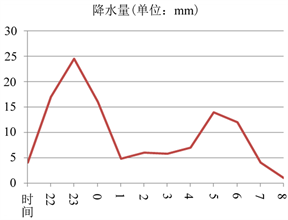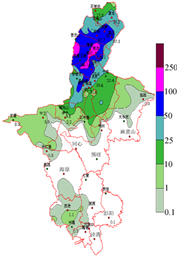(a) (b)

Figure 1. (a) Ningxia precipitation map at 18:00 on July 29 to 8:00 on 30 (unit: mm); (b) YinChuan Railway Station hourly rainfall at 22:00 on July 29 to 8:00 on 30 (unit: mm)

$h=\stackrel{\to }{V}\cdot \nabla \Lambda \stackrel{\to }{V}=\left(u\stackrel{\to }{i}+\upsilon \stackrel{\to }{j}+\omega \stackrel{\to }{k}\right)\cdot \left(\xi \stackrel{\to }{i}+\eta \stackrel{\to }{j}+\varsigma \stackrel{\to }{k}\right)$ (2)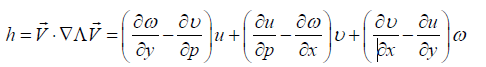(3)

${h}_{p}=-\omega \zeta =-\left(\frac{\partial \upsilon }{\partial x}-\frac{\partial u}{\partial y}\right)\omega$ (4)

3.2. 湿位涡的提出及计算

20世纪40年代，罗斯贝  定义了一个新的物理名词，开辟了暴雨研究的新的方向——位涡。1942埃特  在等熵面上定义了涡度，也称为位势涡度，公式为：

${P}_{E}=\alpha {\stackrel{\to }{\zeta }}_{a}\cdot \nabla \theta$ (5)

$\alpha$ 表示比容， ${\stackrel{\to }{\zeta }}_{\text{a}}$ 表示三维的绝对涡度。这个方程能够将动力、热力作用反映出来，但是位势涡度的公式中没有对水汽影响的计算，难以满足对准确诊断暴雨过程的需求。

$\text{MPV}=-g\left({\zeta }_{p}+f\right)\frac{\partial {\theta }_{se}}{\partial p}+g\left(\frac{\partial \upsilon }{\partial p}\frac{\partial {\theta }_{se}}{\partial x}-\frac{\partial u}{\partial p}\frac{\partial {\theta }_{se}}{\partial y}\right)=const$ (6)

$\text{MPV}1=-g\left({\zeta }_{p}+f\right)\frac{\partial {\theta }_{se}}{\partial p}$ (7)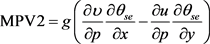(8)

4. 暴雨落区动力诊断

4.1. 垂直螺旋度的诊断

29日08时，正值中心位于 $104˚\text{E}$ 、350 hPa处，强度为 $20×{10}^{-8}\text{hPa}/\text{s}$ ，对流层低层主要为零值区，200 hPa存在一个负值中心，此时银川地区上空处于稳定状态，没有出现降水；29日14时，中低层700 hPa出现正值中心区，强度为 $60×{10}^{-8}\text{hPa}/\text{s}$ ，对应中高层的负值中心，对流不稳定；29日20时，正值中心强度明显增大，达到 $250×{10}^{-8}\text{hPa}/\text{s}$ ，高度发展到400 hPa，并对应中高层强度为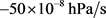的负值中心，已经接近银川，对流不稳定的程度相较上一时刻已经明显加强，处于正值中心边缘影响下的银川站已经出现降水；30日02时，正值中心强度降低，高度下降，中高层依然对应负值区，并且移动到106˚E，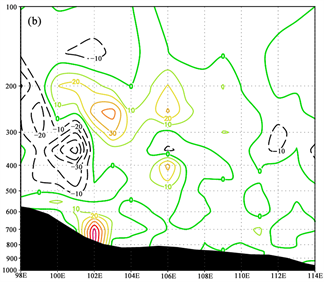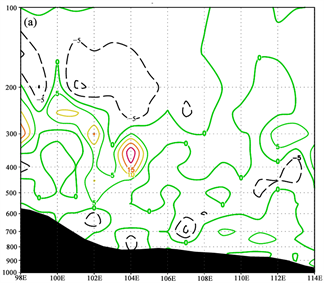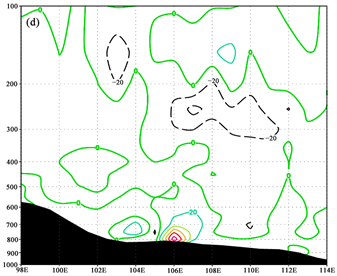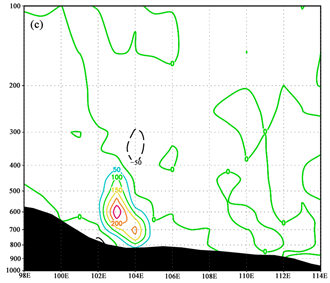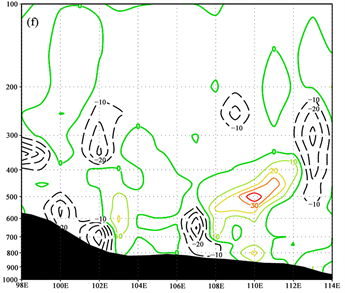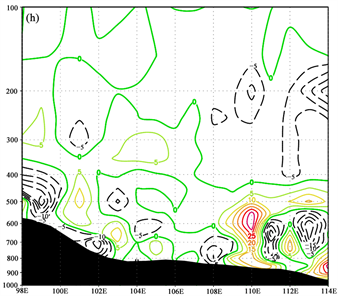Figure 2. Vertical helix along 38˚N vertical profile (unit: ${10}^{-8}\text{hPa}/\text{s}$ ). (a) 08:00 on the 29th; (b) 14:00 on the 29th; (c) 20:00 on the 29th; (d) 02:00 on the 30th; (e) 08:00 on the 30st; (f) 14:00 on the 30th; (g) 20:00 on the 30th; (h) 02:00 on the 31st

4.2. 湿位涡的诊断

4.2.1. 湿正压项的诊断

4.2.2. 湿斜压项的诊断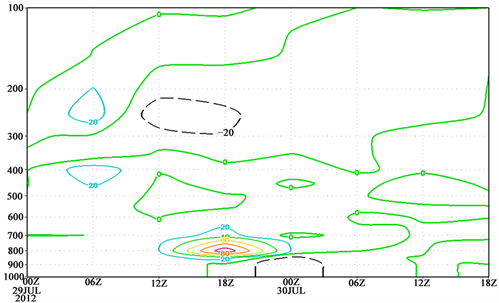Figure 3. Time-height profile along the Yinchuan (106˚N, 38˚E) (unit: ${10}^{-8}\text{hPa}/\text{s}$ )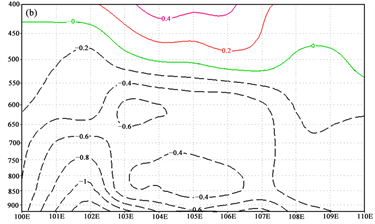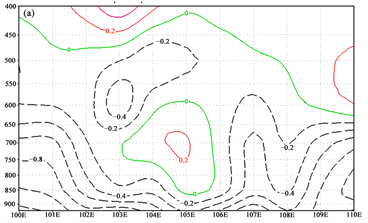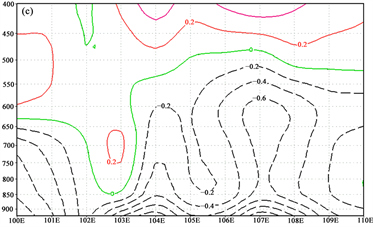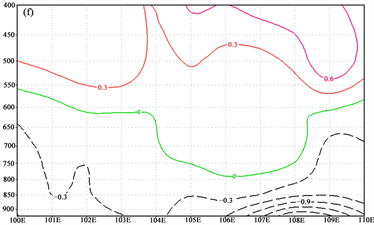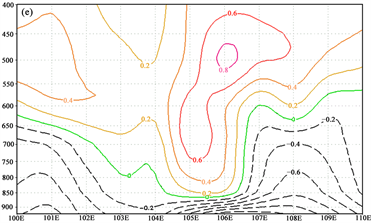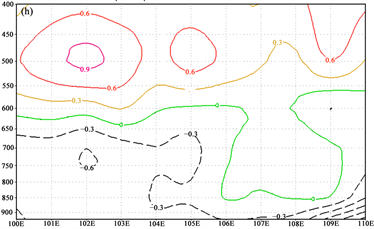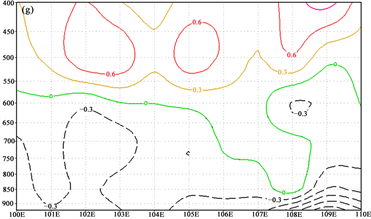Figure 4. Wet positive pressure (MPV1) along 38˚N vertical profile (unit: PVU). (a) 08:00 on the 29th; (b) 14:00 on the 29th; (c) 20:00 on the 29th; (d) 02:00 on the 30th; (e) 08:00 on the 30th; (f) 14:00 on the 30th; (g) 20:00 on the 30th; (h) 02:00 on the 31st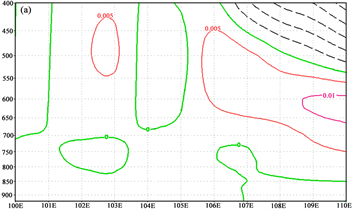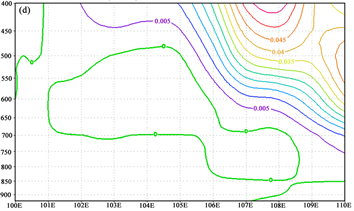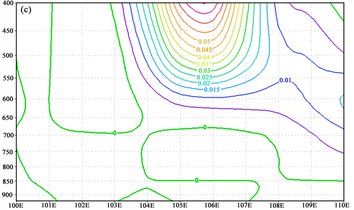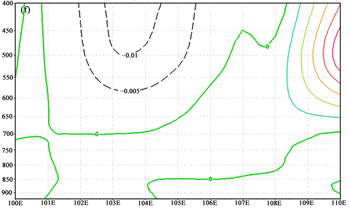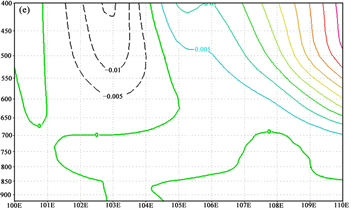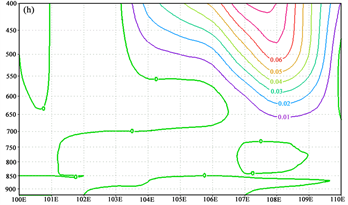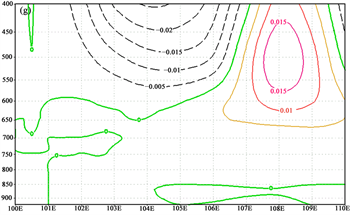Figure 5. Wet oblique (MPV2) along 38˚N vertical profile (unit: PVU). (a) 08:00 on the 29th; (b) 14:00 on the 29th; (c) 20:00 on the 29th; (d) 02:00 on the 30th; (e) 08:00 on the 30th; (f) 14:00 on the 30th; (g) 20:00 on the 30th; (h) 02:00 on the 31st

5. 结论

1) 垂直螺旋度Fz的分析表明：在暴雨时段，对流层低层归螺旋度为正值区、高层负值区，并且随着暴雨的增强，正值区数值增大，负值区数值减小，正值中心也更靠近低层，正、负值区域的大小能够很好的配合实际暴雨的强度。

2) 湿位涡MPV的分析表明：对流层低层湿正压项负值区、中高层湿斜压项正值区相对应，能够体现暴雨的落区，此时对流不稳定、斜压不稳定；湿斜压项数值远小于湿正压项数值，湿斜压项对湿位涡的影响较小，主要依靠湿正压项的作用。

  张虹, 李国平, 王曙东. 西南涡区域暴雨的中尺度滤波分析[J]. 高原气象, 2014, 33(2): 361-371.  杨越奎, 吴宝俊, 沈文梅, 等. “91.7”梅雨锋暴雨的螺旋度分析[J]. 气象学报, 1994, 52(3): 379-384.  陆慧娟. 螺旋度及螺旋度方程的讨论[J]. 气象学报, 2003, 61(6): 684-691.  崔灿. 郑州一次暴雨天气过程诊断分析[J]. 环境科学与技术, 2014, 37(120): 137-141.  留小强, 王田民, 吴宝俊, 等. 湿位涡方程及其应用[J]. 大气科学, 1995, 18(5): 569-575.  高守亭, 雷霆, 周玉淑, 等. 强暴雨系统中湿位涡异常的诊断分析[J]. 应用气象学报, 2002, 13(6): 662-670.  井喜, 李明娟, 王淑云, 等. 青藏高原东侧突发性暴雨的湿位涡分析[J]. 气象, 2007, 33(1): 99-106.  程海霞, 帅克杰, 任璞, 等. 山西南部一次暴雨过程的湿位涡分析[J]. 山西气象, 2005(3): 1-2, 21.  Lilly, D.K. (1986) The Structure, Energetics and Propagation of Rotating Convective Storms. Part I: Energy Exchange with the Mean Flow. Journal of the Atmospheric Sciences, 43, 113-125. https://doi.org/10.1175/1520-0469(1986)043<0113:TSEAPO>2.0.CO;2  张程明. 一次秋季台风暴雨的机制研究[D]: [硕士学位论文]. 兰州: 兰州大学, 2010.  Rossby, C.G. (1940) Planetary Flow Patterns in the Atmosphere. Quarterly Journal of Royal Meteorological Society, 66, 68-87.  Ertel, H. (1942) Einneuer hydrodynamische wirbdsatz. Meteorology. Z. Braunschwei, 59, 277-281.  Bennetts, D.A. and Hoskins, B.J. (1979) Conditional Symmetric Instability—A Possible Explanation for Frontal Rainbands. Quarterly Journal of the Royal Meteorological Society, 105, 945-962.